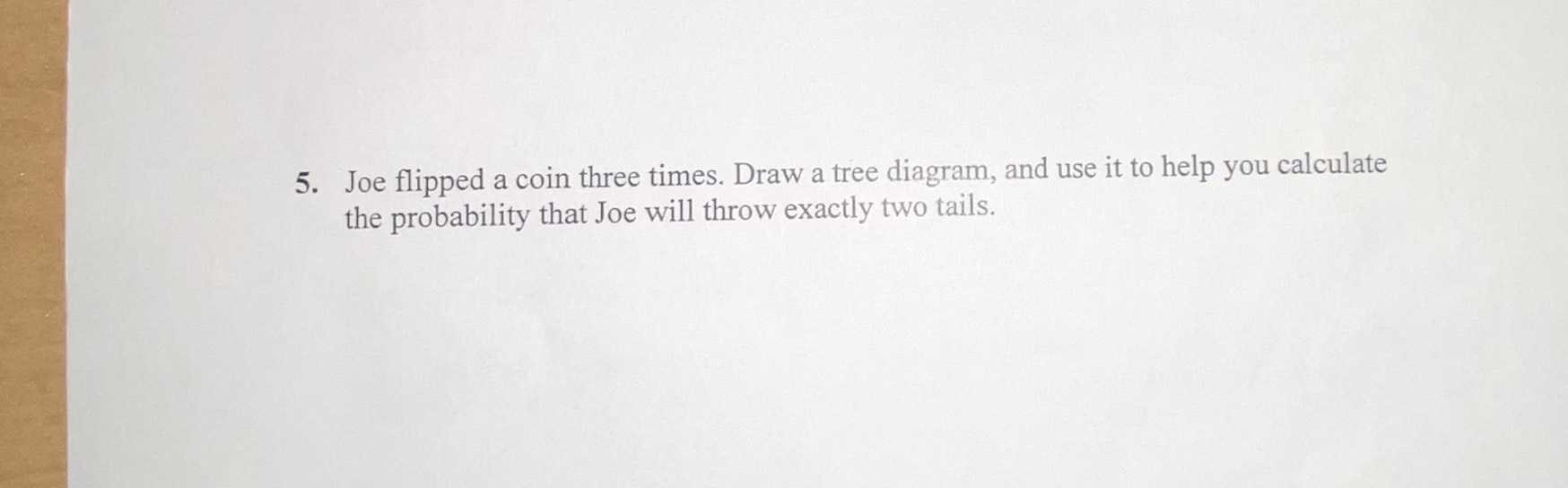### ¿Todavía tienes preguntas de matemáticas?

Pregunte a nuestros tutores expertos
Algebra
Pregunta5. Joe flipped a coin three times. Draw a tree diagram, and use it to help you calculate the probability that Joe will throw exactly two tails.

probability of two tail = $$\frac{3}{8}$$